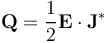Platform: All Platforms Applies to: , Acoustics Module, COMSOL Multiphysics®, MEMS Module, Plasma Module, RF Module, Semiconductor Module, Structural Mechanics Module, Wave Optics Module Versions: All versions

## Problem Description

How does COMSOL Multiphysics handle complex-valued numbers and problems in the frequency domain?

Should I use the RMS, the peak, or the instantaneous value to specify sources in models being solved in the frequency domain, such as electromagnetic or acoustic models?

How can I specify the phase angle?

## Solution

The COMSOL Multiphysics formulation uses complex fields when solving time-harmonic problems in acoustics, electromagnetics, and structural mechanics, or any other physics where the Frequency Domain or Eigenvalue or Eigenfrequency study type is being used. For example, when solving pressure acoustics problems, one is solving for the scalar pressure field: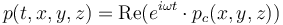where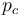is the complex pressure field that you are computing,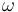is the angular frequency,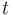is time, and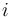is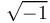.

Whenever you specify a source field in a frequency domain simulation, you can input a complex number (a phasor) such as `pc` where `abs(pc)` is the peak value, and `real(pc)` gives you the instantaneous value at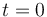.

This applies for any time-harmonic input. So, to define for instance an acoustic pressure, an external current density, a magnetic field, or an electric potential, with an RMS value of 1 and a phase angle of 30°, enter the expression:

`sqrt(2)*exp(i*30[deg])`

or, alternatively:

`sqrt(2)*(cos(30[deg])+i*sin(30[deg]))`

The following functions are useful when dealing with complex-valued numbers:

`real(a)` Returns the real component of the argument.

`imag(a)` Returns the imaginary component of the argument.

`abs(a)` Returns the magnitude of the complex-valued argument.

`arg(a)` Returns the phase.

`conj(a)` Returns the complex conjugate.

`realdot(a,b)` Returns the dot product of the two input arguments. The result is identical to `real(a*conj(b))` but also will define the correct partial derivatives.

In many cases, especially in electromagnetics, a complex-valued vector field (such as the electric field) is of interest. So, the electric field in a 3D model would have components: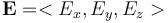For such vector fields there will be a norm defined, in terms of the vector dotted with its complex-conjugate: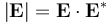When evaluating results, keep in mind that this norm is equivalent to the magnitude of the vector field, and the RMS value would be a factor of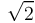less.

In electromagnetic problems, there is also usually a cycle-averaged heating computed. So, for example, for a frequency-domain electric currents problem the resistive heating term is defined as: